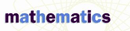# Field Properties - Research Article from Macmillan Science Library: Mathematics

This encyclopedia article consists of approximately 4 pages of information about Field Properties.
 This section contains 965 words (approx. 4 pages at 300 words per page) View a FREE sampleDavid Hilbert, a famous German mathematician (1862–1943), called mathematics the rules of a game played with meaningless marks on paper. In defining the rules of the game called mathematics, mathematicians have organized numbers into various sets, or structures, in which all the numbers satisfy a particular group of rules. Mathematicians call any set of numbers that satisfies the following properties a field: closure, commutativity, associativity, distributivity, identity elements, and inverses.

## Determining a Field

Consider the set of non-negative even numbers: {0, 2, 4, 6, 8, 10, 12,…}. To determine whether this set is a field, test to see if it satisfies each of the six field properties.

Closure. When any two numbers from this set are added, is the result always a number from this set? Yes, adding two non-negative even numbers will always result in a non-negative even number. The set of non-negative even numbers is therefore closed under addition.

Is the set...

(read more)

 This section contains 965 words (approx. 4 pages at 300 words per page) View a FREE sampleMore summaries and resources for teaching or studying Field Properties.
CopyrightsField Properties from Macmillan. Copyright © 2001-2006 by Macmillan Reference USA, an imprint of the Gale Group. All rights reserved.